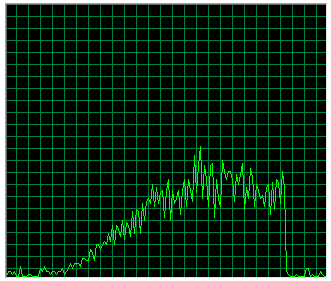[This article was first published on Blog - Data Science and the fst Package, and kindly contributed to R-bloggers]. (You can report issue about the content on this page here)
Want to share your content on R-bloggers? click here if you have a blog, or here if you don't.

The fst package uses the xxHash algorithm for internal hashing of (meta-)data. With method hash_fst the user now has direct access to this extremely fast hashing algorithm.

# Hashing

Hashing is used to map data of any size to data of a (usually much smaller) fixed size. That’s very useful for creating fast lookup tables, a digital data signature or in cryptography. The fst package hashes all meta-data that is stored in the fst format and will support optional hashing of columnar data as well in the near future. Storing hashes in the format greatly adds to the security and stability as errors in the format can easily be detected by comparing hashes.

For hashing in fst the excellent and speedy xxHash algorithm is used, developed by Yann Collet. Method hash_fst provides direct access to the xxHash library and also includes a multi-threaded variant that hashes at extreme speeds.

To demonstrate the hash_fst interface, we use a 93 MB file downloaded from Kaggle.

``````# file downloaded from https://www.kaggle.com/stackoverflow/so-survey-2017
sample_file <- "survey_results_public.csv"

# file size (in MB)
1e-6 * file.size(sample_file)
``````
``````##  93.09709
``````

To calculate the hash value of data contained in the raw_vec vector, we use:

``````library(fst)

hash_fst(raw_vec)
``````
``````##   1853499107 -1914678989
``````

The return value is a length two integer vector because the hashing algorithm is actually a 64-bit hashing algorithm (and a single integer occupies 32 bits in memory). Based on the already fast xxHash algorithm, the speed of the multi-threaded hash implementation in fst is pretty extreme:

``````threads_fst(8)

hash_timing <- microbenchmark(
hash_fst(raw_vec),
times = 1000
)

# hashing speed (GB/s)
as.numeric(object.size(raw_vec)) / median(hash_timing\$time)
``````
``````hash_timings[Threads == 8, Speed]
``````
``````##  46.72303
``````

That’s a hashing speed of more than 46 GB/s!

# Dependency on number of cores

With a small benchmark, we can reveal how the multi-threaded hashing depends on the selected number of cores:

``````library(data.table)

# result table
bench <- as.list(rep(1, 20))

hash_timing <- microbenchmark(
hash_fst(raw_vec),
times = 1000
)

}

bench <- rbindlist(bench)
``````

The computer used for the benchmark has two Xeon E5 CPU’s (@2.5GHz) with 10 physical cores each. The results are displayed below:Figure 1: hashing speed vs the number of cores used for computation

Using more than 8-10 threads doesn’t help performance. It’s clear that the Xeon is hitting other boundaries than computational speed, such as the maximum memory bandwidth and thread- or CPU synchronization issues. That’s also confirmed by the less than 100 percent pressure on CPU resources during the benchmark:Figure 2: CPU resources used during benchmark